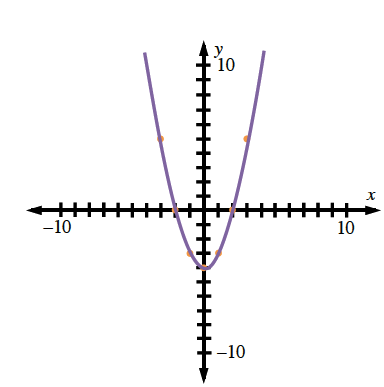### Home > AC > Chapter 4 > Lesson 4.1.3 > Problem4-22

4-22.

For the rule $y=x^2−4$, calculate the $y$-values that complete the table below. Plot the points and connect them on a complete graph on graph paper. What does your graph look like?

 IN (x) OUT (y) $-3$ $-2$ $-1$ $0$ $1$ $2$ $3$

Substitute the values for $x$ into the given equation to find the values of $y$.

 IN (x) OUT (y) $-3$ $-2$ $-1$ $0$ $1$ $2$ $3$ $\boldsymbol{5}$ $\boldsymbol{0}$ $\boldsymbol{-3}$ $\boldsymbol{-4}$

Graph the points and connect them with a continuous curve.